# 5) The following integrals compute the volume of a solid with a known cross-section. For each...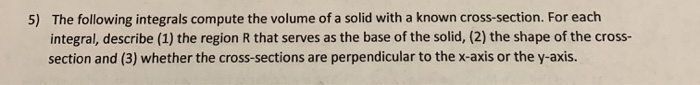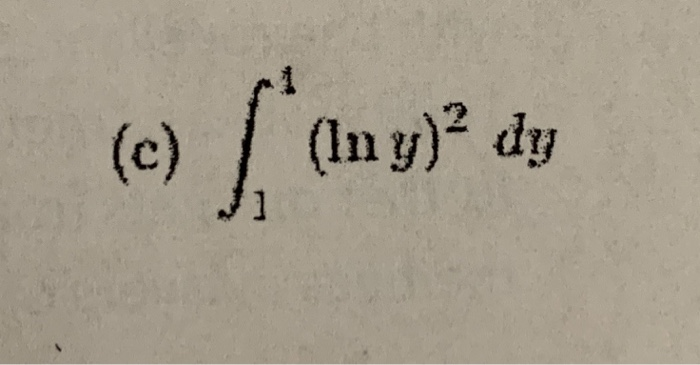5) The following integrals compute the volume of a solid with a known cross-section. For each integral, describe (1) the region R that serves as the base of the solid, (2) the shape of the cross- section and (3) whether the cross-sections are perpendicular to the x-axis or the y-axis.
(c) (Iny)? dy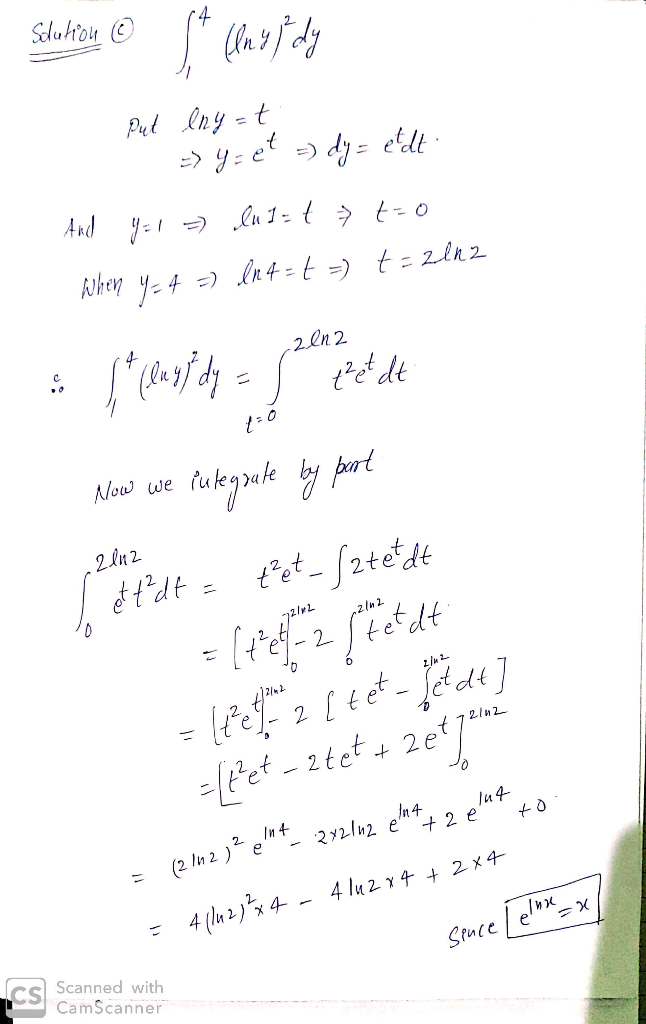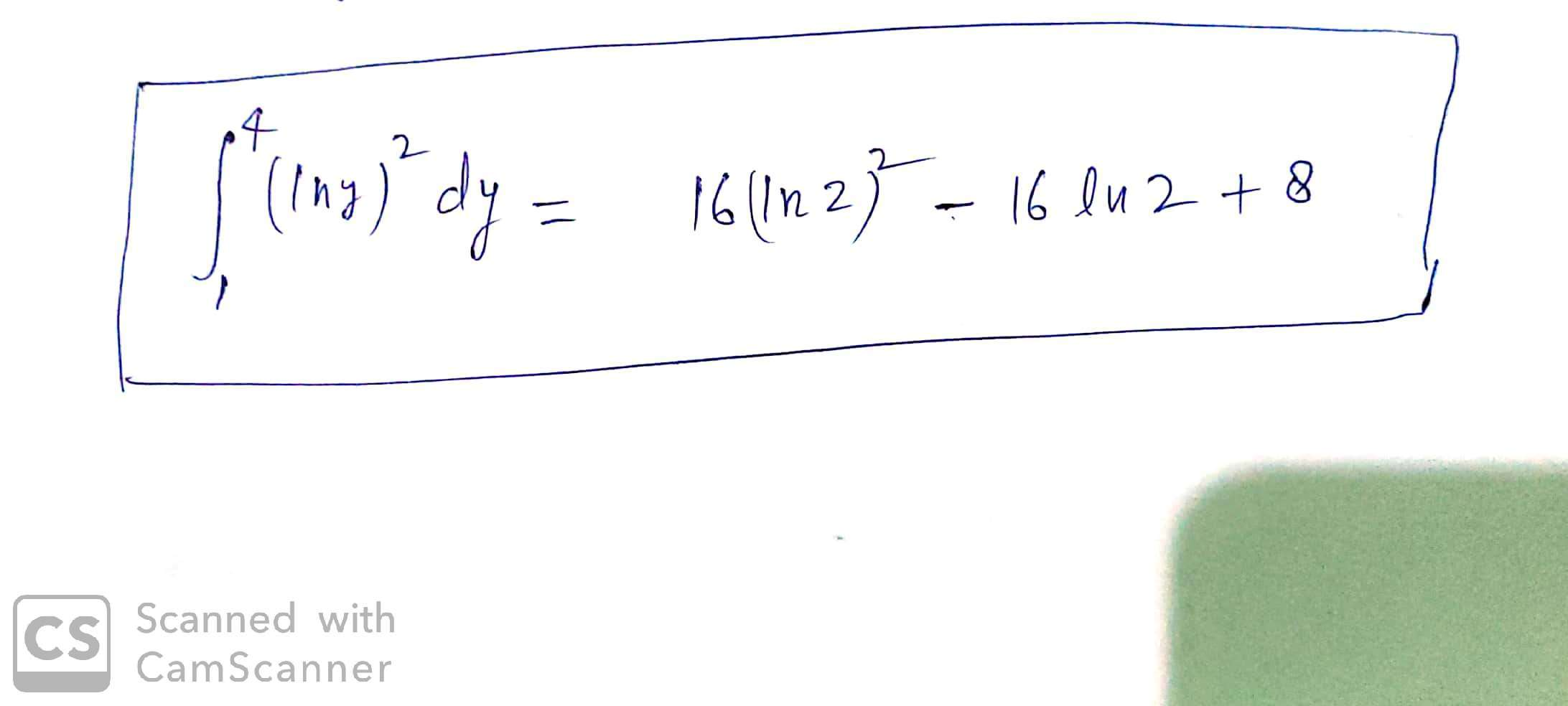#### Earn Coin

Coins can be redeemed for fabulous gifts.

Similar Homework Help Questions
• ### Determine the volume of a solid by integrating a cross-section with a triangle Question The solid...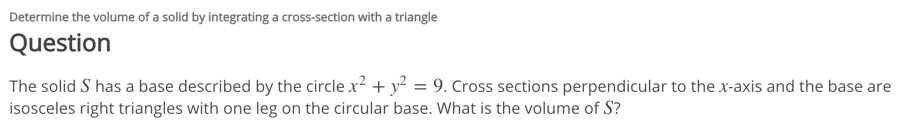Determine the volume of a solid by integrating a cross-section with a triangle Question The solid S has a base described by the circle x' + y2 = 9. Cross sections perpendicular to the x-axis and the base are isosceles right triangles with one leg on the circular base. What is the volume of S?

• ### Use calculus to find the volume of the following solid S: The base of S is the parabolic region {(x,y)1x2 < y < 1 } . cross-sections perpendicular to the y- axis are squares. Volume - Use...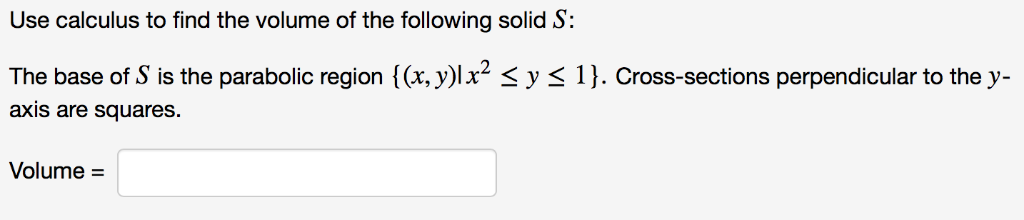Use calculus to find the volume of the following solid S: The base of S is the parabolic region {(x,y)1x2 < y < 1 } . cross-sections perpendicular to the y- axis are squares. Volume - Use calculus to find the volume of the following solid S: The base of S is the parabolic region {(x,y)1x2

• ### Problem 5: 6 pts) The base of a solid is the region in the ry-plane bounded by 2+2 32 and y and is shown below. Cro...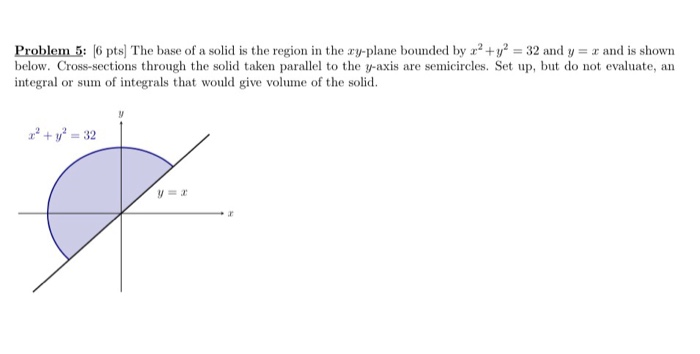Problem 5: 6 pts) The base of a solid is the region in the ry-plane bounded by 2+2 32 and y and is shown below. Cross-sections through the solid taken parallel to the y-axis are semicircles. Set up, but do not evaluate, an integral or sum of integrals that would give volume of the solid. 32 Problem 5: 6 pts) The base of a solid is the region in the ry-plane bounded by 2+2 32 and y and is shown...

• ### 5. Let R be the region bounded by the graph of, y Inr + 1) the line y 3, and the line x - 1. (a)S...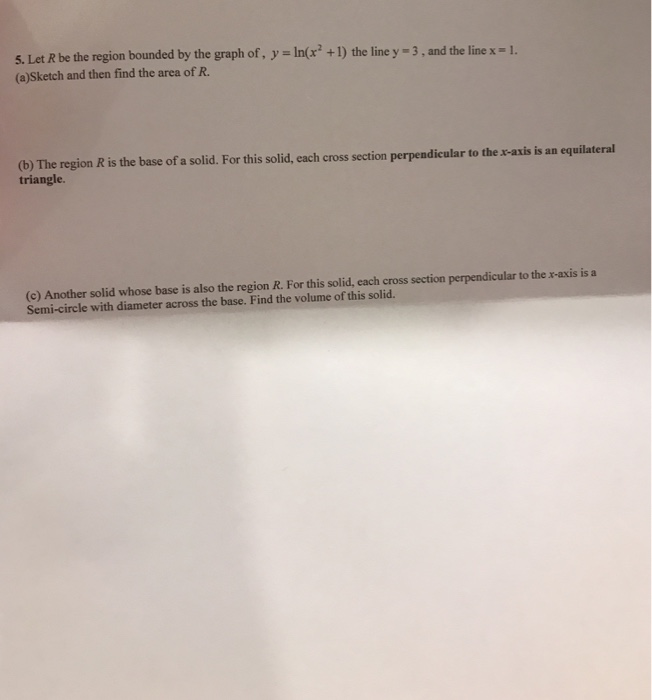5. Let R be the region bounded by the graph of, y Inr + 1) the line y 3, and the line x - 1. (a)Sketch and then find the area of R (b) The region R is the base of a solid. For this solid, each cross section perpendicular to the x-axis is an equilateral triangle. (c) Another solid whose base is also the region R. For this solid, each cross section perpendicular to the x-axis is a Semi-circle...

• ### The last one was incorrect Use the general slicing method to find the volume of the...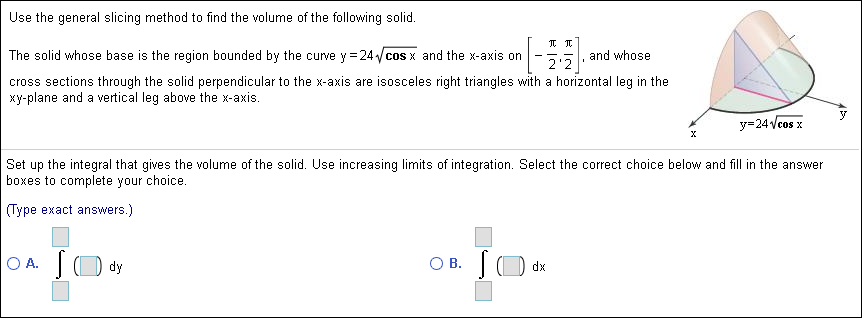The last one was incorrect Use the general slicing method to find the volume of the following solid. The solid whose base is the region bounded by the curve y 24cos x and the x-axis on and whose 2'2 cross sections through the solid perpendicular to the x-axis are isosceles right triangles with a horizontal leg in the xy-plane and a vertical leg above the x-axis. y 24Vcos x Set up the integral that gives the volume of the solid....

• ### Determine the Volume of a Solid by Integrating a Cross-Section With a Circle or Semicircle Question...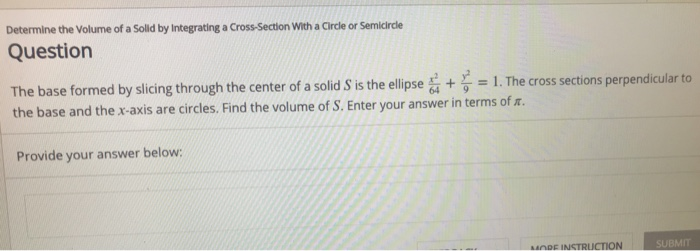Determine the Volume of a Solid by Integrating a Cross-Section With a Circle or Semicircle Question The base formed by slicing through the center of a solid S is the ellipse + y,-1. The cross sections pe the base and the x-axis are circles. Find the volume of S. Enter your answer in terms of r. 64 9 Provide your answer below: MODE INSTRUCTION MI

• ### For each problem, find the volume of the solid that results when the region enclosed by...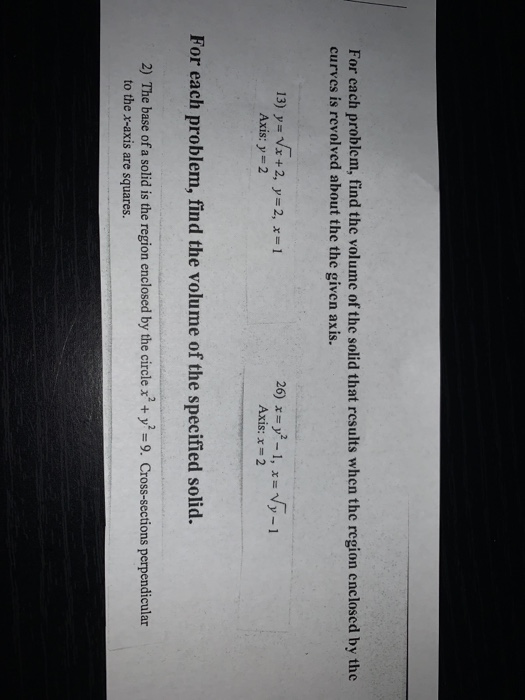For each problem, find the volume of the solid that results when the region enclosed by the curves is revolved about the given axis. For each problem, find the volume of the solid that results when the region enclosed by the curves is revolved about the the given axis. 13) y= Vx+2, y=2, x=1 Axis: y = 2 26) *= y2 - 1, x= Vy-1 Axis: x = 2 For each problem, find the volume of the specified solid. 2)...

• ### 2. Find the volume of a solid whose cross section, perpendicular to the x -axis, has area given by x3 for each x in...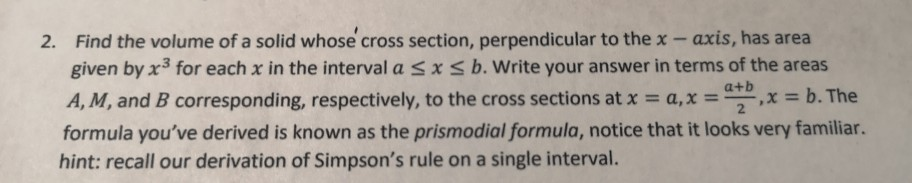2. Find the volume of a solid whose cross section, perpendicular to the x -axis, has area given by x3 for each x in the interval a sx s b. Write your answer in terms of the areas A, M, and B corresponding, respectively, to the cross sections at x x -b. The a+b formula you've derived is known as the prismodial formula, notice that it looks very familiar. hint: recall our derivation of Simpson's rule on a single interval....

• ### Consider a solid whose base is the region bounded by the curves y = (−x^2) + 3 and y = 2x − 5, wi...

Consider a solid whose base is the region bounded by the curves y = (−x^2) + 3 and y = 2x − 5, with cross-sections perpendicular to the y-axis that are squares. a) Sketch the base of this solid. b) Find a Riemann sum which approximates the volume of this solid. c) Write a definite integral that calculates this volume precisely. (Do not need to calculate the integral)

• ### cannot figure out how to write the integrals for this problem #2 1. If glx) -2x and fx) - , find the area of the region enclosed by the two graphs. Show a work for full credit. (4 pts) 2. A:12-...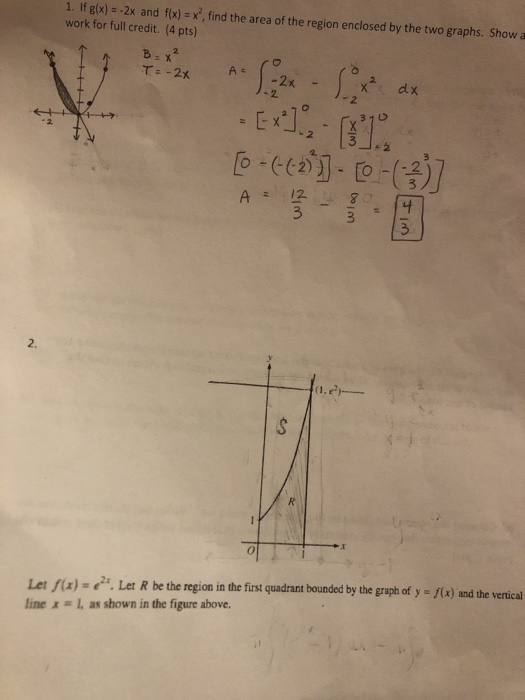cannot figure out how to write the integrals for this problem #2 1. If glx) -2x and fx) - , find the area of the region enclosed by the two graphs. Show a work for full credit. (4 pts) 2. A:12-80% 3 3 2 Let fix)-. Let R be the region in the first quadrant bounded by the gruph of y - f(x) and the vertical line x # l, as shown in the figure above. (a) Write but do...